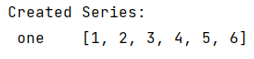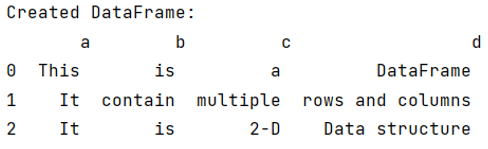# What is the difference between a Pandas Series and a DataFrame?

Learn about the differences between Pandas Series and a DataFrame in Python.
Submitted by Pranit Sharma, on June 04, 2022

## Difference between a Pandas Series and a DataFrame

Both DataFrame and series are the two main data structure of pandas library. Series in pandas contains a single list which can store heterogeneous type of data, because of this, series is also considered as a 1-dimensional data structure. On the other hand, DataFrame is a 2-dimensional data structure which contains multiple lists of heterogeneous type of data. DataFrame can contain multiple series or it can be considered as a collection of series.

When we analyse a series, each value can be considered as a separate row of a single column, whereas when we analyse a DataFrame, we have multiple columns and multiple rows.

Both series and DataFrame can be created either with list or with the help of a dictionary, in case of series, there will be only one key in the dictionary but there may be multiple keys in case of DataFrame.

Note

To work with pandas, we need to import pandas package first, below is the syntax:

```import pandas as pd
```

Let us understand by implementing both Series and DataFrame,

## Python Series Example

```# Importing pandas package
import pandas as pd

# Create dictionary
d = {'one':[1,2,3,4,5,6]}

# Create series
ser = pd.Series(d)

# Display series
print("Created Series:\n",ser)
```

### Output

The output of the above program is:## Python DataFrame Example

```# Importing pandas package
import pandas as pd

# Create dictionary
d = {
'a':['This','It','It'],
'b':['is','contain','is'],
'c':['a','multiple','2-D'],
'd':['DataFrame','rows and columns','Data structure']
}

# Create DataFrame
df = pd.DataFrame(d)

# Display DataFrame
print("Created DataFrame:\n",df)
```

### Output

The output of the above program is: